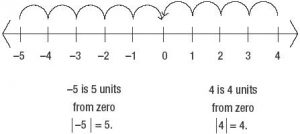# Absolute Values and Inequalities###### An Illusion!
July 29, 2016###### GMAT Probability
November 2, 2016Absolute values

The absolute value represents the distance from zero on a number line. Mathematically absolute of X is written as |X|. Absolute value is always positive because it disregards the direction (whether negative or positive). For example, the number 7 is exactly 7units away from 0, so the absolute value of 7 equals 7 (i.e.|7|=7).  To find the absolute value of -7, look at the real number line, number -7 is also exactly 7 units away from 0 (i.e.|-7|=7). In general

|positive| = positive

|negative| = positive

Absolute value bars (i.e.| |) are conceptually a measure of the distance of the expression inside the bar from zero.

Solving Equations with Absolute Values:

An equation with an absolute value has two solutions and it is solved by considering two circumstances.

Example: |2x-4|>6

1. Write the problem without absolute sign and solve the inequalities.

2x-4>6
2x > 10
x >5

2. Write the problem without absolute value sign, reverse the inequality and negate the value not under absolute value and solve the inequalities.

2x-4 < -6
2x<-6+4
2x<-2
x<-1

The interval notation for this solution is -1>x>5.  Multiplying inequality range by negative, inequality signs are reversed and this can also be written as 1<x<-5.

Inequalities

A mathematical statement that uses one of the following inequality signs is called an inequality.

< Less than
> Greater than
≤ Less than or equal to
≥ Greater than or equal to

Inequalities can involve variables and are similar to equations, except that the two sides are related by one of the inequality signs instead of the equality sign used in equations.

The procedure used to solve a linear inequality is similar to that used to solve a linear equation, which is to simplify the inequality by isolating the variable on one side of the inequality, using the following two rules.

Rule 1: When the same constant is added to or subtracted from both sides of an inequality, the direction of the inequality is preserved and the new inequality is equivalent to the original.

Example:

-5 ≤ A < 6
-5+2 ≤ A < 6+2
-3 ≤ A <8

Rule 2: When both sides of the inequality are multiplied or divided by the same nonzero constant, the direction of the inequality is preserved if the constant is positive but the direction is reversed if the constant is negative. In either case, the new inequality is equivalent to the original.

Example:

-5 ≤ A < 6

Multiply the above inequality by -2 on the both sides

-2 x -5 ≤ A < 6 x -2
-12<A ≤ 10